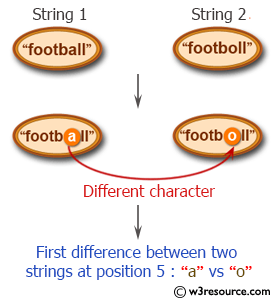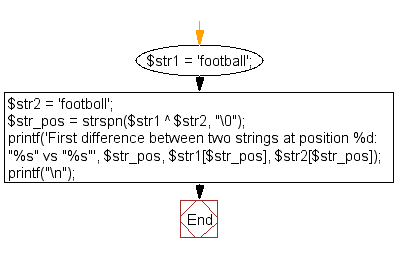﻿ PHP String Exercise: Find the first character that is different between two strings - w3resource# PHP String Exercises: Find the first character that is different between two strings

## PHP String: Exercise-11 with Solution

Write a PHP script to find the first character that is different between two strings.

String1 : 'football'
String2 : 'footboll'

Pictorial Presentation:Sample Solution:

PHP Code:

``````<?php
\$str1 = 'football';
\$str2 = 'footboll';
\$str_pos = strspn(\$str1 ^ \$str2, "\0");
printf('First difference between two strings at position %d: "%s" vs "%s"',
\$str_pos, \$str1[\$str_pos], \$str2[\$str_pos]);
printf("\n");
?>
```
```

Sample Output:

```First difference between two strings at position 5: "a" vs "o"
```

Flowchart :PHP Code Editor:

Have another way to solve this solution? Contribute your code (and comments) through Disqus.

What is the difficulty level of this exercise?

Test your Programming skills with w3resource's quiz.

﻿

## PHP: Tips of the Day

Members of objects or classes can be accessed using the object operator (->) and the class operator (::).

Example:

```class MyClass {
public \$a = 1;
public static \$b = 2;
const C = 3;
public function d() { return 4; }
public static function e() { return 5; }
}
\$object = new MyClass();
var_dump(\$object->a); // int(1)
var_dump(\$object::\$b); // int(2)
var_dump(\$object::C); // int(3)
var_dump(MyClass::\$b); // int(2)
var_dump(MyClass::C); // int(3)
var_dump(\$object->d()); // int(4)
var_dump(\$object::d()); // int(4)
var_dump(MyClass::e()); // int(5)
\$classname = "MyClass";
var_dump(\$classname::e()); // also works! int(5)
```

Note that after the object operator, the \$ should not be written (\$object->a instead of \$object->\$a). For the class operator, this is not the case and the \$ is necessary. For a constant defined in the class, the \$ is never used.

Also note that var_dump(MyClass::d()); is only allowed if the function d() does not reference the object:

```class MyClass {
private \$a = 1;
public function d() {
return \$this->a;
}
}
\$object = new MyClass();
var_dump(MyClass::d()); // Error!
```

This causes a 'PHP Fatal error: Uncaught Error: Using \$this when not in object context'

These operators have left associativity, which can be used for 'chaining':

```class MyClass {
private \$a = 1;

\$this->a += \$a;
return \$this;
}

public function get() {
return \$this->a;
}
}
\$object = new MyClass();
```

These operators have the highest precedence (they are not even mentioned in the manual), even higher that clone. Thus:

```class MyClass {
private \$a = 0;
\$this->a += \$a;
return \$this;
}
public function get() {
return \$this->a;
}
}
\$o1 = new MyClass();
var_dump(\$o1->get()); // int(2)
var_dump(\$o2->get()); // int(2)
```

The value of \$o1 is added to before the object is cloned!

Note that using parentheses to influence precedence did not work in PHP version 5 and older (it does in PHP 7):

```// using the class MyClass from the previous code
\$o1 = new MyClass();
\$o2 = (clone \$o1)->add(2); // Error in PHP 5 and before, fine in PHP 7
var_dump(\$o1->get()); // int(0) in PHP 7
var_dump(\$o2->get()); // int(2) in PHP 7
```